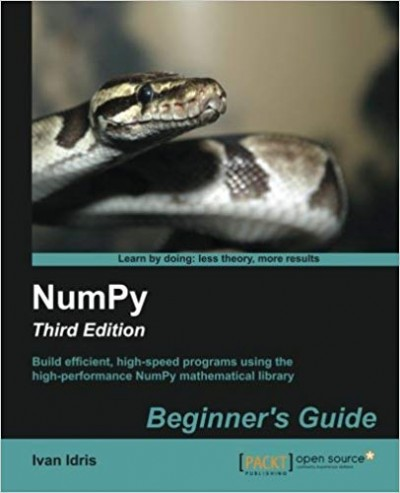# NumPy: Beginner's Guide, 3rd Edition – pdf – 电子书免费下载In today’s world of science and technology, it’s all about speed and flexibility. When it comes to scientific computing, NumPy tops the list. NumPy will give you both speed and high productivity. This book will walk you through NumPy with clear, step-by-step examples and just the right amount of theory. The book focuses on the fundamentals of NumPy, including array objects, functions, and matrices, each of them explained with practical examples. You will then learn about different NumPy modules while performing mathematical operations such as calculating the Fourier transform, finding the inverse of a matrix, and determining eigenvalues, among many others. This book is a one-stop solution to knowing the ins and outs of the vast NumPy library, empowering you to use its wide range of mathematical features to build efficient, high-speed programs.

Who This Book Is For

This book is for the scientists, engineers, programmers, or analysts looking for a high-quality, open source mathematical library. Knowledge of Python is assumed. Also, some affinity, or at least interest, in mathematics and statistics is required. However, I have provided brief explanations and pointers to learning resources.

What You Will Learn

• Install NumPy, matplotlib, SciPy, and IPython on various operating systems
• Use NumPy array objects to perform array operations
• Familiarize yourself with commonly used NumPy functions
• Use NumPy matrices for matrix algebra
• Work with the NumPy modules to perform various algebraic operations
• Test NumPy code with the numpy.testing module
• Plot simple plots, subplots, histograms, and more with matplotlib

ISBN:1785281968

## 锦瑟无端五十弦，一弦一柱思华年

### 觉得文章有用就打赏一下文章作者

#### 支付宝扫一扫打赏#### 微信扫一扫打赏